### Calculated Field

• date 14th March, 2020 |
• by Prwatech |

Calculated fields are one of the most powerful features of Tableau which allows to create new data from existing data set. It can be used to make new measures such as ratios or even new dimensions such as segments. Calculated fields can also be used with any data type, aggregations and functions, as well as logical operators.

Let’s learn how to make Calculated Fields in the following steps. Here, we have used Samples EU Superstore data which is provided by Tableau by default.

Click on the little arrow beside Dimensions as shown by the arrow in the diagram given below. Then click on Create Calculated Field.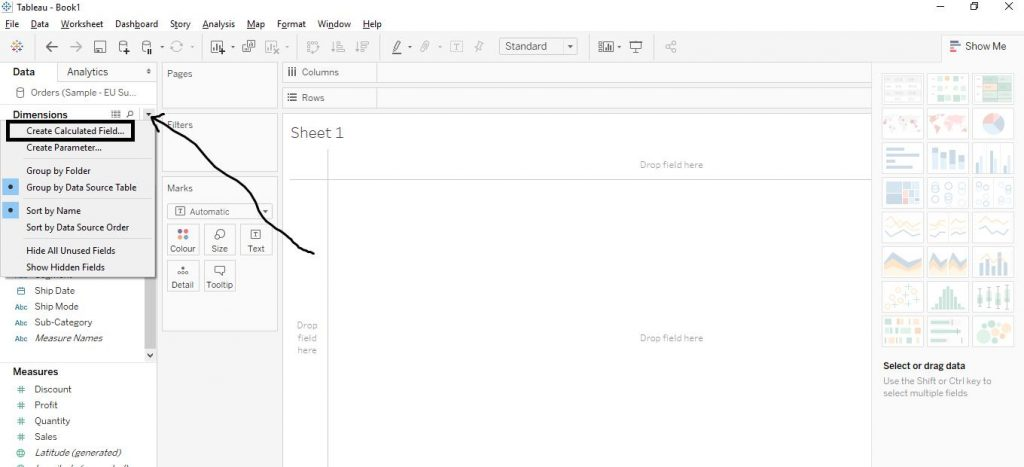On clicking the Create Calculated Field, the following window appears as shown by the diagram below. On the right hand side, the functions list appears and on clicking on them, the definition of the function is given. On top left, the name of the Calculated Field is to be put and below it, the formulae are written.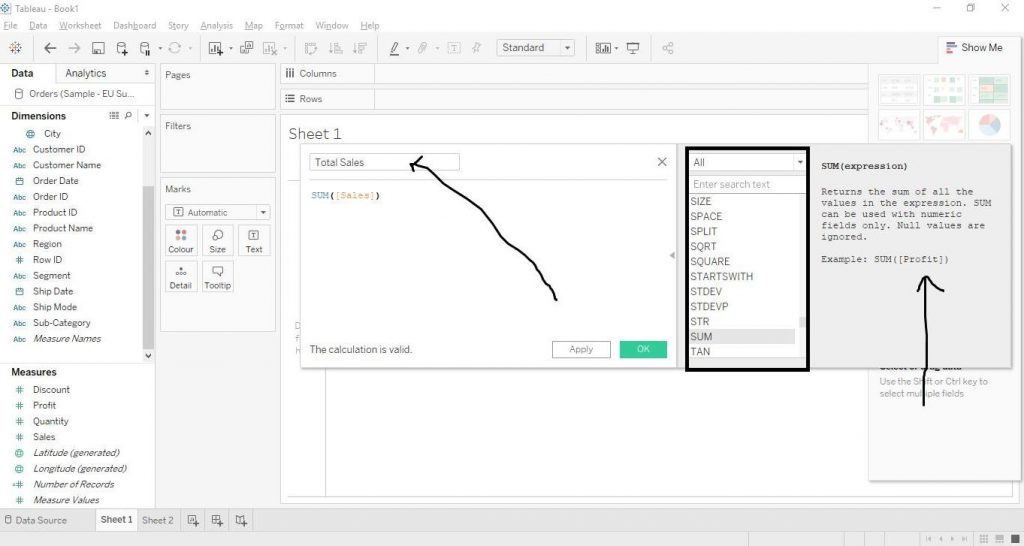As the figure above shows, we have written the function for Total Sales as SUM ([Sales]).Then click on OK. And then the Calculated Field gets created as shown in the diagram below.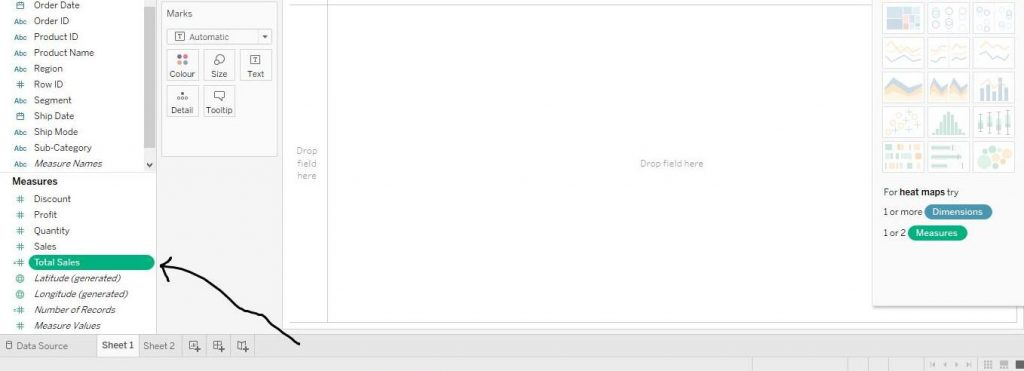We can check the value of Total Sales by dragging it from Measures Section to Text in the Marks Card. The diagram below demonstrates the step.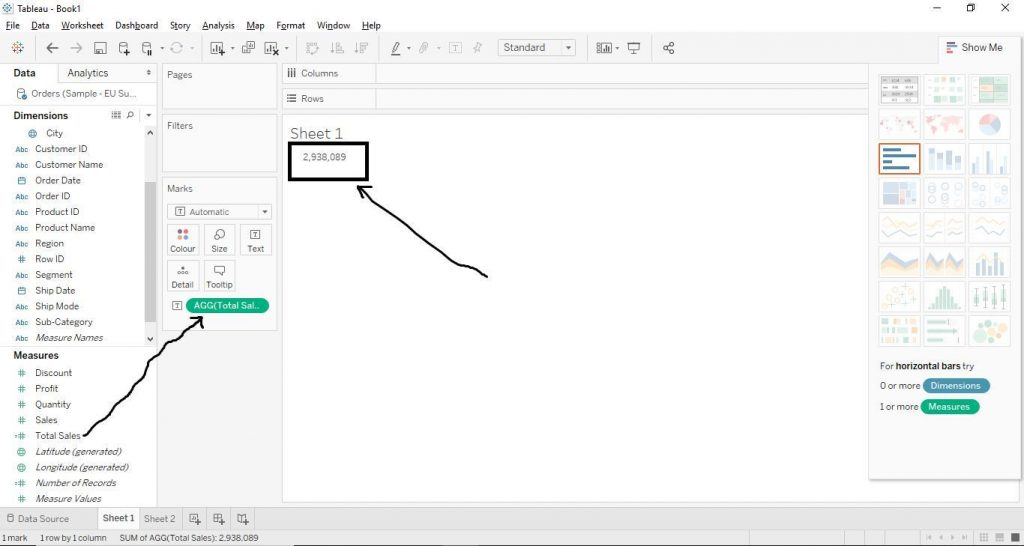We can also edit the Calculated Field created. Select the Calculated Field, here, Total Sales. Then click on the little arrow beside it and click on Edit as shown by the arrow in the diagram.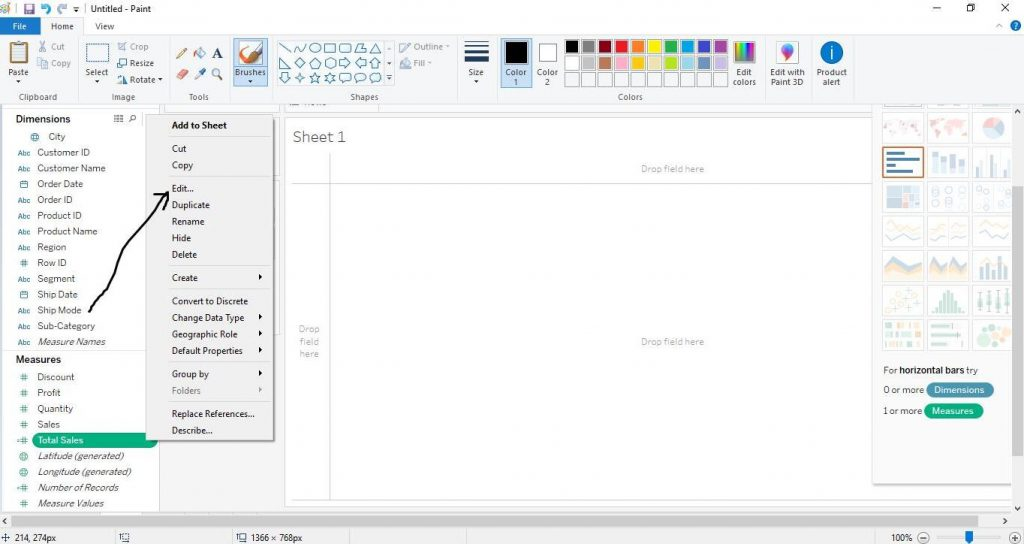Then change the name of the Calculated Field, here, we have changed Total Sales to Average Sales. And also, change the function written to AVG ([Sales]) and click on OK.  The diagram below demonstrates the step.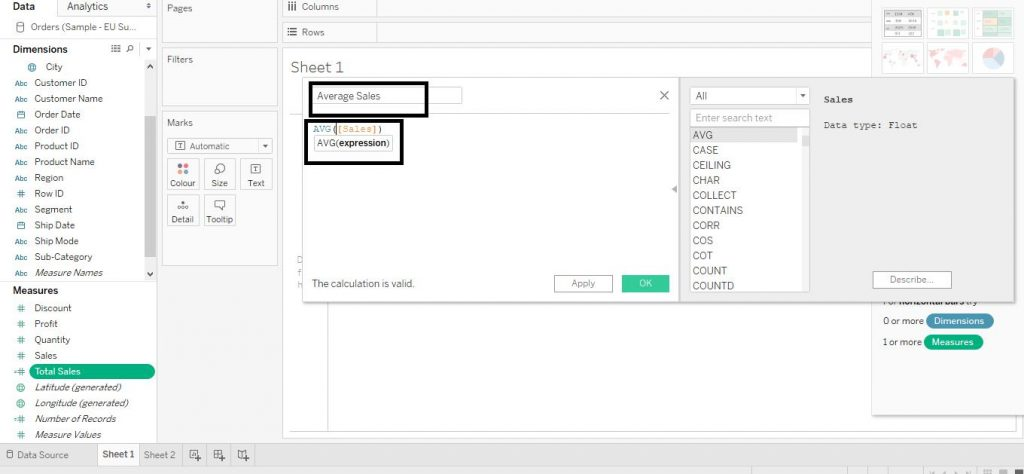The Calculated Field Total Sales gets changed to Average Sales along with the change of calculations in the functions as well.  Then in order to check the value of Average Sales, drag Average Sales from Measures to Text in the Marks Card. The diagram below shows the following step.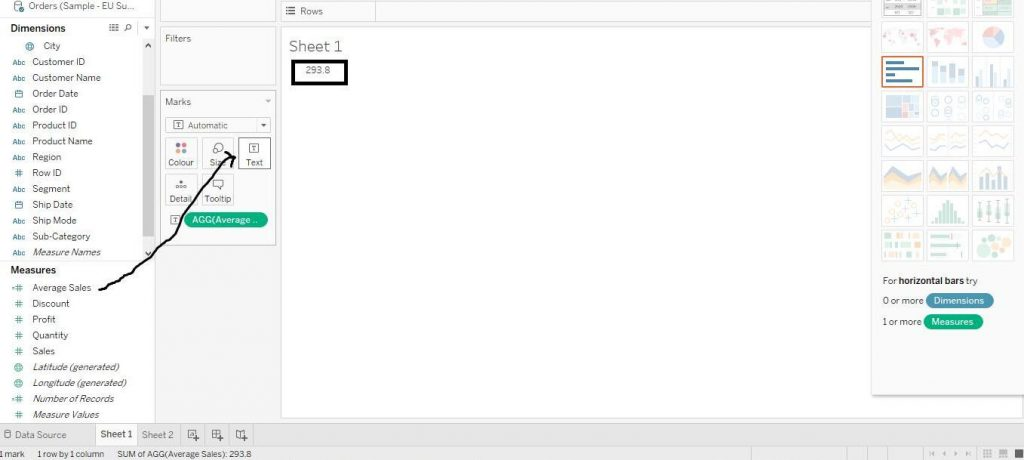### Quick Support